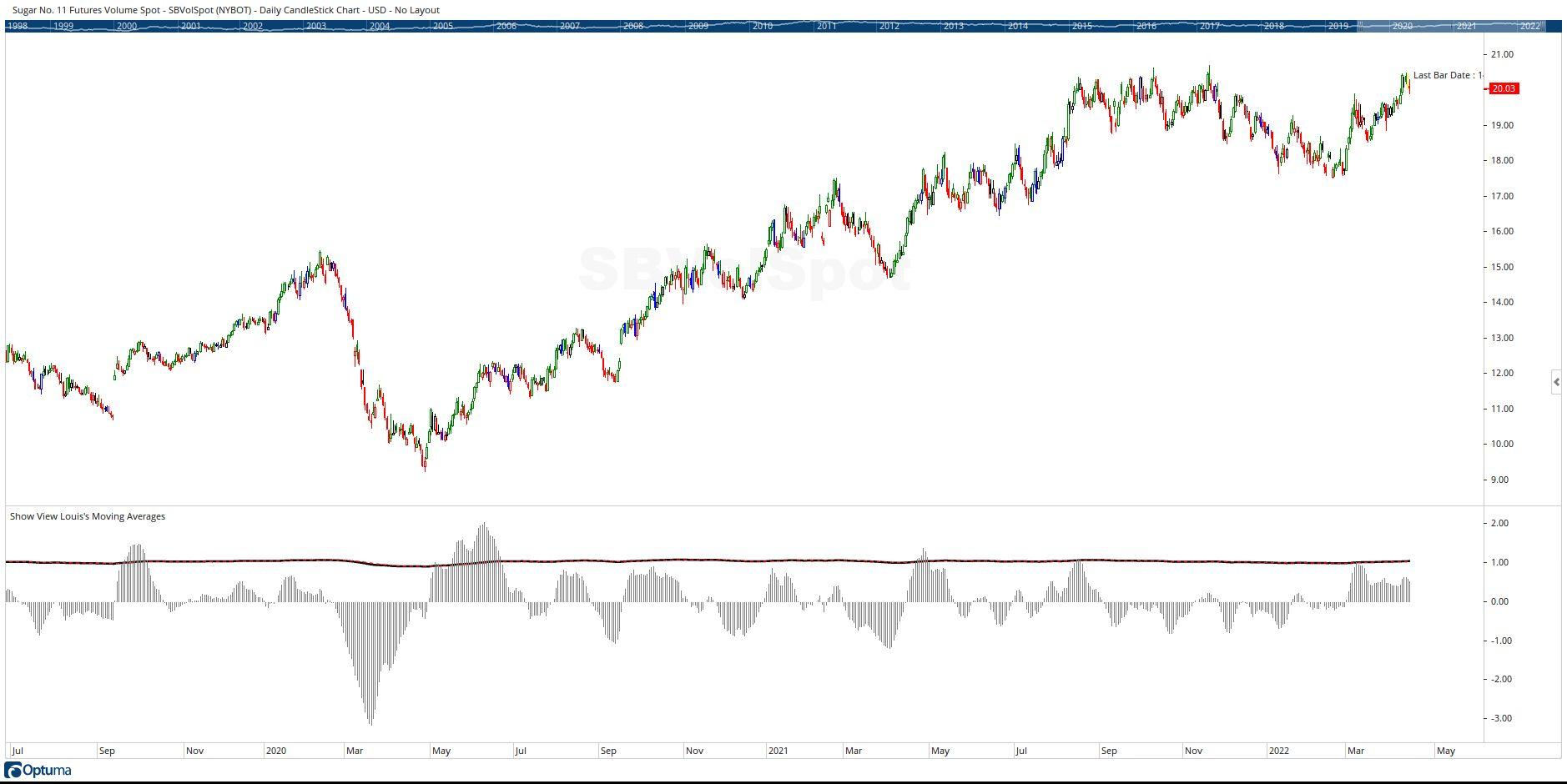Select Page

# Reply To: Pring’s Trend Deviation Script

Optuma Forums Optuma Scripting Pring’s Trend Deviation Script Reply To: Pring’s Trend Deviation Script

#67957

Hi Louis,

Your line Plot3 = (Plot1 – Plot2) ; is trying to subtract one array from another array. Plots are arrays and that doesn’t work. You need to do the subtraction on the creation of Plot3:

Plot3 = (MA1 / MA2) – (MA(Plot1, BARS=9, CALC=Close, STYLE=Exponential) );

Note that the values for Plot 3 are very small compared to Plot1 and Plot2, so to see the values all on one view you would need to multiply Plot3 by some factor, say 100,  like this:

Plot3 = ((MA1 / MA2) – (MA(Plot1, BARS=9, CALC=Close, STYLE=Exponential) ) )* 100;

which will produce the result shown in the following screenshot:Cheers

Trevor